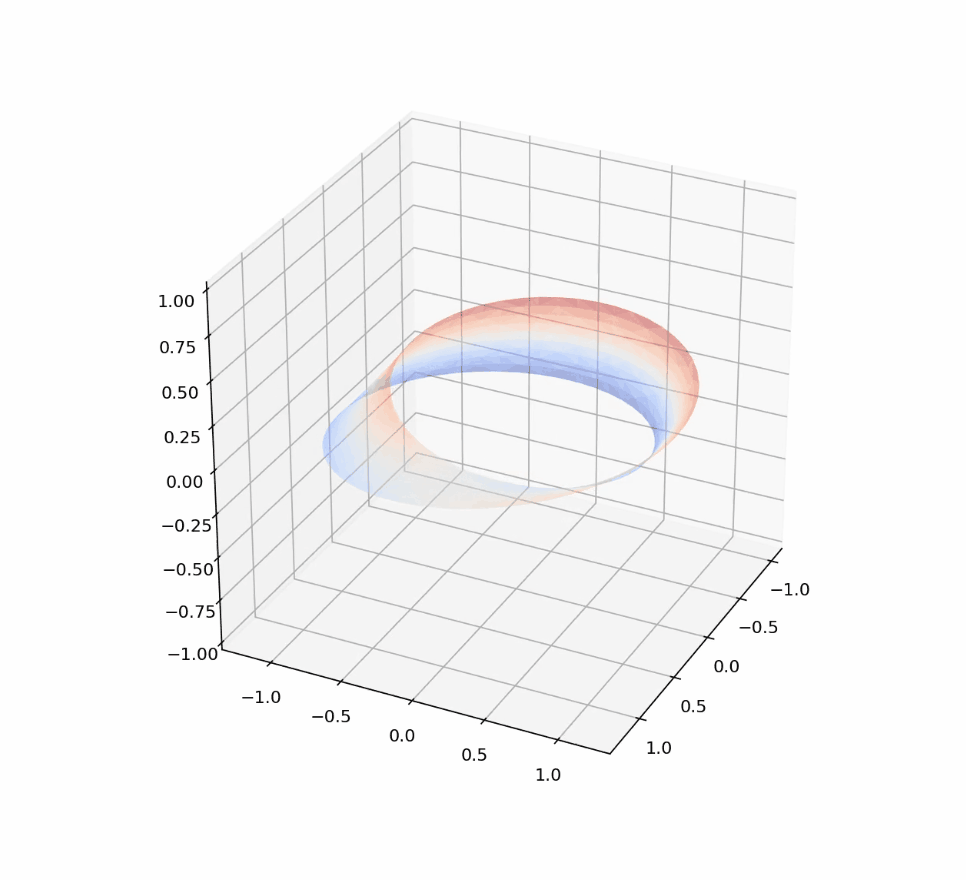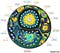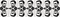# Language Processing with Recurrent Models

## Bidirectional RNNs, Encoding, Word Embedding and Tips# What’s SimpleRNN?

`from keras.layers import SimpleRNN`
• All of the successive outputs aka the states
• Just the last state
`from keras.models import Sequentialfrom keras.layers import Embedding, SimpleRNNmodel = Sequential() model.add(Embedding(10000…`

# Convolutional Models for Sequential Data

## Easing Into Recurrent Neural Networks

Translation Invariance

A convolutional model can learn a certain pattern in the lower right area, then after that point detect it anywhere on the image.

Spatial Hierarchy

A convolutional model can learn patterns in a hierarchical fashion, much like we do. The first layers will learn relatively simple patterns, like horizontalness and verticalness etc. Then the second layers will put these together to learn such things as corners. And so on with each new layer.

# Working Understanding of Convolutional Models

## Creating, Preprocessing, Data Augmentation, Feature Extraction, Fine Tuninghttps://www.graphcore.ai/posts/what-does-machine-learning-look-like

# What does a CNN model look like in code?

`from keras import layers from keras import models  seq_model= models.Sequential() seq_model.add(layers.Conv2D(32, (3, 3), activation='relu', input_shape=(28, 28, 1))) seq_model.add(layers.MaxPooling2D((2, 2))) seq_model.add(layers.Conv2D(64, (3, 3), activation='relu')) seq_model.add(layers.MaxPooling2D((2, 2))) seq_model.add(layers.Conv2D(128, (3, 3), activation='relu'))`
`from keras import modelsseq_model= models.Sequential()`
`from keras.models import Sequential, Modelnon_seq_model = Model(input_tensor, output_tensor)`
`from keras import layersseq_model.add(layers.Conv2D(32, (3, 3), activation='relu', input_shape=(28, 28, 1)))`
• Here the `input_shape `is (28, 28, 1), which takes an input tensors of shape (`image_height`, `image_width`, `image_channels`). …

# Summary of the Previous Article

• Language
• Strings
• Symbols
• Sets
• Operations
• Properties
• Rules
• Classes of Languages

# Natural Language Tool Kit 3.5

## Search Functions, Statistics, Pronoun Resolutionhttps://www.newyorker.com/culture/culture-desk/living-in-alan-turings-future

# Basics:

• Goal
• Input & Output
• Encoding & Decoding
• Architecture
• Regularization
• Validation

# Code:

• Import
• Model Definition
• K-Fold Validation
• Validation
• Final Model

# Input & Output

1. CRIM per capita crime rate by town
2. ZN proportion of residential land zoned for lots over 25,000 sq.ft.
3. INDUS proportion of non-retail business acres per town
4. CHAS Charles River dummy variable (= 1 if tract bounds river; 0…## Jake Batsuuri

I write about software && math